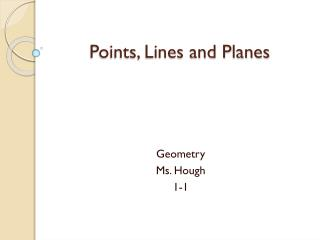DownloadDownload PresentationPoints, Lines and Planes

# Points, Lines and Planes

Download Presentation## Points, Lines and Planes

- - - - - - - - - - - - - - - - - - - - - - - - - - - E N D - - - - - - - - - - - - - - - - - - - - - - - - - - -
##### Presentation Transcript

1. Points, Lines and Planes Geometry Ms. Hough 1-1

2. Vocabulary • A point is simply a location • A line is made up of points and has no thickness or width. • Points on the same line are said to be collinear. • A plane is a flat surface made up of points • Points that lie on the same plane are said to be coplanar.

3. Lets make this easier…

4. Example 1 • Use the figure to name each of the following: • A line containing point A • A plane containing point C E D C B N A

5. Example 2 • Draw and label a figure for each relationship • Lines GH and JK intersect at L for G(-1, -3), H(2, 3), J(-3, 2) and K(2, -3) on a coordinate plane. Point M is coplanar with these points but not collinear with GH or JK.

6. Example 3- Interpret Drawings • How many planes appear in this figure? • Name three points that arecoplanar. • Are points G, A, R and E coplanar? Explain. • At what point do EF and AB intersect? D G C P E B A

7. Modeling Intersecting Planes • Label one index card as Q and another as R. • Hold the two index cards together and cut a slit halfway through both cards • Hold the cards so that the slits meet and insert one card into the slit of the other. Use tape to hold the cards together. • Where the two cards meet models a line. Draw the line and label two points, C and D, on the line. Answer the following Questions • Draw a point F on your bodel so that it lies in Q but not in R. Can F lie on DC? Explain. • If point H lies in both Q and R, where would it lie? Draw point H on your model. • Draw a sketch of your model on paper. Label all points, lines and planes.